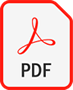×
00:00
/

✕ Course Content

#### Introduction

The Caribbean Secondary Education Certificate (CSEC) Mathematics examination is a crucial component of the secondary education curriculum in the Caribbean.
The examination, which is typically taken by students in their final year of secondary school, tests their knowledge and understanding of a wide range of mathematical concepts, including algebra, geometry, trigonometry, and calculus.

One of the key strengths of the CSEC Mathematics examination is that it not only tests students' ability to solve mathematical problems, but also their ability to think critically and apply their mathematical knowledge to real-world situations. This is important because mathematics is not just a subject to be studied for its own sake, but is also an essential tool for solving problems in many different fields, including science, engineering, finance, and business. Another strength of the CSEC Mathematics examination is that it is designed to be accessible to a wide range of students, regardless of their background or level of mathematical ability. This is achieved by providing a variety of question types, such as multiple choice, short answer, and extended response, as well as a range of difficulty levels. This ensures that students are able to demonstrate their knowledge and understanding of mathematical concepts, regardless of their learning style or aptitude.

In preparing for the CSEC Mathematics examination, students should focus on developing their problem-solving skills, as well as their ability to think critically and apply mathematical concepts to real-world situations. This can be achieved through regular practice, using a variety of different types of problems and question formats, as well as by seeking help and guidance from teachers and other experts when needed.

##### Topics include:

• Numbers and Number Operations:
This section covers topics such as whole numbers, fractions, decimals, ratio and proportion, and percentage.
• Algebra and Algebraic Techniques:
This section covers topics such as algebraic expressions, equations, inequalities, and functions.
• Algebra and Algebraic Techniques:
This section covers topics such as algebraic expressions, equations, inequalities, and functions.
• Geometry and Trigonometry:
This section covers topics such as points, lines, angles, polygons, circles, and trigonometry.
• Measurement:
This section covers topics such as length, mass, capacity, time, and temperature.
• Statistics and Probability:
This section covers topics such as data collection, representation, and interpretation, as well as probability.
• Calculus:
This section covers topics such as limits, differentiation, and integration.
Additionally, the syllabus also includes a section on the use of technology in mathematics.
The syllabus also includes the development of critical thinking, problem-solving, and reasoning skills, as well as the use of technology in mathematics. It is designed to provide students with a solid foundation in mathematics and to prepare them for further studies in the field.

#### Past PapersMay 2020January 2022January 2021July 2021January 2020July 2021